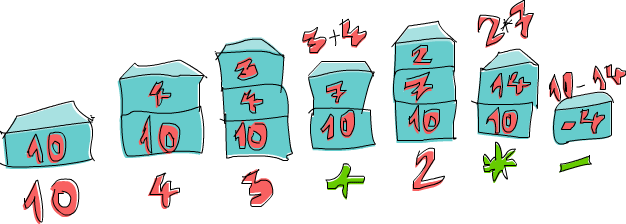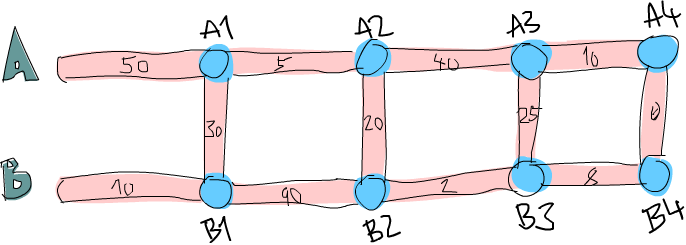# 函数式地思考来解决问题

## 运算逆波兰表示法(Reverse Polish notation form)``````小建议：在你去实作函数之前，先想一下你会怎么宣告这个函数的型别能够帮助你厘清问题。在 Haskell 中由于我们有够强的型别系统，光从函数的宣告就可以得到许多信息。
````````````import Data.List

solveRPN :: (Num a) => String -> a
solveRPN expression = head (foldl foldingFunction [] (words expression))
where   foldingFunction stack item = ...
``````

``````import Data.List

solveRPN :: (Num a) => String -> a
solveRPN = head . foldl foldingFunction [] . words
where   foldingFunction stack item = ...
``````

``````solveRPN :: (Num a, Read a) => String -> a
solveRPN = head . foldl foldingFunction [] . words
where   foldingFunction (x:y:ys) "*" = (x * y):ys
foldingFunction (x:y:ys) "+" = (x + y):ys
foldingFunction (x:y:ys) "-" = (y - x):ys
foldingFunction xs numberString = read numberString:xs
``````

``````ghci> solveRPN "10 4 3 + 2 * -"
-4
ghci> solveRPN "2 3 +"
5
ghci> solveRPN "90 34 12 33 55 66 + * - +"
-3947
ghci> solveRPN "90 34 12 33 55 66 + * - + -"
4037
ghci> solveRPN "90 34 12 33 55 66 + * - + -"
4037
ghci> solveRPN "90 3 -"
87
``````

``````import Data.List

solveRPN :: String -> Float
solveRPN = head . foldl foldingFunction [] . words
where   foldingFunction (x:y:ys) "*" = (x * y):ys
foldingFunction (x:y:ys) "+" = (x + y):ys
foldingFunction (x:y:ys) "-" = (y - x):ys
foldingFunction (x:y:ys) "/" = (y / x):ys
foldingFunction (x:y:ys) "^" = (y ** x):ys
foldingFunction (x:xs) "ln" = log x:xs
foldingFunction xs "sum" = [sum xs]
foldingFunction xs numberString = read numberString:xs
``````

``````ghci> solveRPN "2.7 ln"
0.9932518
ghci> solveRPN "10 10 10 10 sum 4 /"
10.0
ghci> solveRPN "10 10 10 10 10 sum 4 /"
12.5
ghci> solveRPN "10 2 ^"
100.0
``````

``````ghci> solveRPN "43.2425 0.5 ^"
6.575903
``````

## 路径规划``````50
10
30
5
90
20
40
2
25
10
8
0
````````````也许你会问如果先在 B1 跨到道路 A 然后走到 A2 的情况呢？我们已经考虑过了从 B1 到 A1 的情况，所以我们不需要再把他考虑进去。
``````

``````data Node = Node Road Road | EndNode Road
``````

``````data Node = Node Road (Maybe Road)
``````

``````data Section = Section { getA :: Int, getB :: Int, getC :: Int } deriving (Show)
``````

``````当然我们也可以用一个 tuple ``(Int, Int, Int)`` 来代表一个 section。使用 tuple 对于一些简单的情况是比较方便，但对于比较复杂的情况定义自己的 algebraic data type 会比较好。他让型别系统获得比较多的信息。``(Int, Int, Int)`` 毕竟也可能被使用在定义三维空间中的一个矢量，只用 tuple 让我们可能把这两种情形混杂起来使用。如果我们用 ``Section`` 跟 ``Vector`` 的话就不会不小心搞混了。
``````

``````heathrowToLondon :: RoadSystem
heathrowToLondon = [Section 50 10 30, Section 5 90 20, Section 40 2 25, Section 10 8 0]
``````

``````data Label = A | B | C deriving (Show)
type Path = [(Label, Int)]
``````

``````[(B,10),(C,30),(A,5),(C,20),(B,2),(B,8)]
``````

``````提示：把 ``(Path, Path) -> Section -> (Path, Path)`` 当作 left fold 用的二元函数，fold 要求的型态是 ``a -> b -> a``。
``````
``````roadStep :: (Path, Path) -> Section -> (Path, Path)
roadStep (pathA, pathB) (Section a b c) =
let priceA = sum \$ map snd pathA
priceB = sum \$ map snd pathB
forwardPriceToA = priceA + a
crossPriceToA = priceB + b + c
forwardPriceToB = priceB + b
crossPriceToB = priceA + a + c
newPathToA = if forwardPriceToA <= crossPriceToA
then (A,a):pathA
else (C,c):(B,b):pathB
newPathToB = if forwardPriceToB <= crossPriceToB
then (B,b):pathB
else (C,c):(A,a):pathA
in  (newPathToA, newPathToB)
````````````ghci> roadStep ([], []) (head heathrowToLondon)
([(C,30),(B,10)],[(B,10)])
``````

``````优化小技巧：当我们写 ``priceA = sum \$ map snd pathA`` 的时候。我们是在计算每步的成本。如果我们实作 ``roadStep`` 成 ``(Path, Path, Int, Int) -> Section -> (Path, Path, Int, Int)`` 的话就可以不必那么做。其中的整数型别代表 A 跟 B 上的最小成本。
``````

``````optimalPath :: RoadSystem -> Path
in  if sum (map snd bestAPath) <= sum (map snd bestBPath)
then reverse bestAPath
else reverse bestBPath
``````

``````ghci> optimalPath heathrowToLondon
[(B,10),(C,30),(A,5),(C,20),(B,2),(B,8),(C,0)]
``````

``````groupsOf :: Int -> [a] -> [[a]]
groupsOf 0 _ = undefined
groupsOf _ [] = []
groupsOf n xs = take n xs : groupsOf n (drop n xs)
``````

``````import Data.List

main = do
contents <- getContents
let threes = groupsOf 3 (map read \$ lines contents)
roadSystem = map (\[a,b,c] -> Section a b c) threes
pathString = concat \$ map (show . fst) path
pathPrice = sum \$ map snd path
putStrLn \$ "The best path to take is: " ++ pathString
putStrLn \$ "The price is: " ++ show pathPrice
``````

``````50
10
30
5
90
20
40
2
25
10
8
0
``````

``````\$ cat paths.txt | runhaskell heathrow.hs
The best path to take is: BCACBBC
The price is: 75
``````